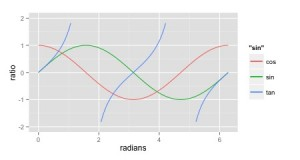# Category Archives: Math# Equivalent of Numpy’s Clip function in R

Numpy’s clip function is a handy function that brings all data in a series into a range. For example, in machine learning, it is common to have activation functions that take a continuous range of values and bring them to a range like 0 to 1, or -1 to 1.

In my case, I was working on some quality control charts that have control limits. In this case, control limits could be calculated to be below 0, but should never be. I was working in R. R has a function max() that can take two values and return the max. So by applying that function over a series, you make make sure each value was at least 0. But it felt a bit cumbersome compared to clip. min() and max() are not vectorized in R. That makes sense, because you may want the max or min of the entire series, so it makes sense that you can pass a series or many values, and get a single answer.

Note that in my use case, the second value is a scalar. But it could be a vector of the same size.

I discovered the pmin and pmax functions which can clip a single bound, or be combined to approximate the clip function. Here’s a gist showing plots of how this works:

# Markov Chain Simulation

I’ve been reading up on Markov chains and related concepts. On the wikipedia page there is an example of a 2 state Markov process. I decided to simulate it in R and plot the mean of the means.

Quick Code example here: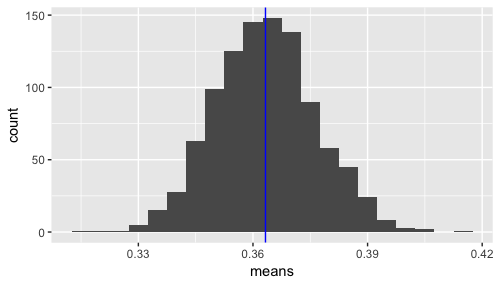The mean of means (of state e) is close to .36. If you take .3 * .36 + .4 * (1-.36), you get .364, so this seems to make sense. Note that I’m weighting the switching to e percentage based on the percentage of being in that state in the first place.

# The Math of Machine Learning

One of the challenges of data science in general is that it is a multi-disciplinary field. For any given problem, you may need skills in data extraction, data transformation, data cleaning, math, statistics, software engineering, data visualization, and the domain. And that list likely isn’t inclusive.

One of the first questions when it comes to machine learning in specific, is “how much math do I need to know?”

This is where I would recommend you start, to get the most value for your time:

• Matrix Multiplication (Subject: Linear Algebra)
• Probability (Subject: Statistics)
• Normal Distributions (Subject: Statistics)
• Bayes Theorem (Subject: Statistics)
• Linear Regression (Subject: Statistics)

Of course you will run across other math needs, but I think the above list represents the foundation.

If you need places to get started with those topics, check out Kahn Academy, Coursera, or your location library.

For more on machine learning, check out other posts such as ML in R, Linear Algebra in R, and ML w/XGBoost.

# Presentation on Linear Algebra in R

At our January meeting, I presented on Linear Algebra basics in R. I have been taking the Andrew Ng’s Stanford Machine Learning course. That course primarily uses Matlab (or Octave, and open source equivalent), and machine learning involves manipulating and calculating with matrices. Naturally, being an R person, I have been working with some of the techniques in R.

In order to limit the scope of the talk, I focused on matrices, vectors and basic operations with them. There is a practical example that uses a machine learning algorithm, but it’s just to show how R handles a more involved equation with matrices. The talk is not an attempt to teach machine learning.

The slides are available here, and comments or suggestions are welcome.

# Mapping Functions in R

I have been buffing up on some areas of Math that I felt rusty in. One of the tools I was using was my old TI-82 calculator I used in high school.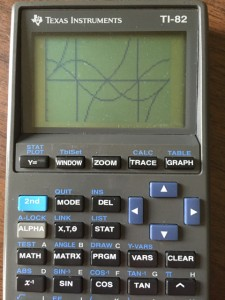I even got the TI Connect software working where I could download screenshots, etc.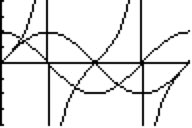You can put in data sets (see screen cap below), and do some graphing and some statistical analysis.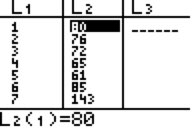However, mapping functions is a more straight forward use of the calculator. Which got me thinking… How does one do that in R?

I did a little digging. With the traditional R graphics and plotting functions, you would use curve() to draw a function. It works fine, but I like to use ggplot2 when I can.

Turns out ggplot2 supports this well. The following code sample maps the same functions I was mapping on the calculator (sin, cos, and tan from 0 to 2pi radians).

And the resulting graph is…